# How does Shader Graph connect the polar filter effect of PS?

PS polar coordinate effect, as shown

I want to know how to use Shader Graph to connect this effect. I tried his polar coordinate node, but got this effect, he is not what I want

I hope someone will help me solve it. My English is not very good

2 Likes

Converts the value of input UV to polar coordinates. In mathematics, the polar coordinate system is a two-dimensional coordinate system in which each point on a plane is determined by a distance from a reference point and an angle from a reference direction.

The resulting effect is that the x channel of the input to UV is converted to a distance value from the point specified by the value of input Center and the y channel of same input is converted to the value of an angle of rotation around that point.

These values can be scaled by the values of inputs Radial Scale and Length Scale respectively.
From the node wiki.

Try length scale to 1``````void Unity_PolarCoordinates_float(float2 UV, float2 Center, float RadialScale, float LengthScale, out float2 Out)
{
float2 delta = UV - Center;
float angle = atan2(delta.x, delta.y) * 1.0/6.28 * LengthScale;
}
``````

This thread might be useful to you

1 Like

Change the length ratio to 1 and that’s it.

:混淆:混淆:

2 Likes

Oh thats because of how the shader calculates, the texture orientation is important.
Example(Ignore the quality of the iamges, ive actually took same from above xD):
With this image: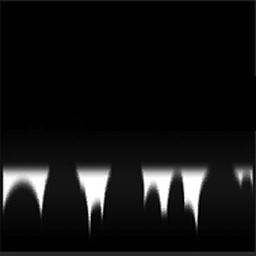This is the result: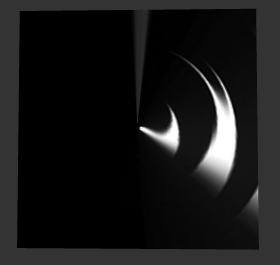And with this same but rotated 90º: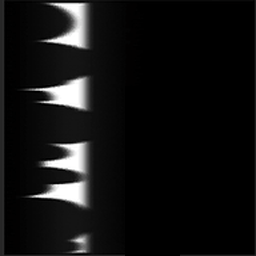Result: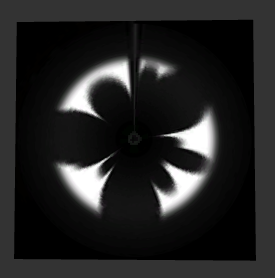And if you want it by the other side: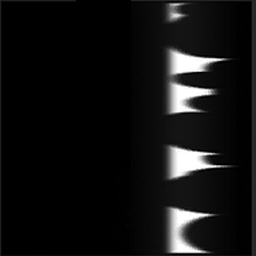Result: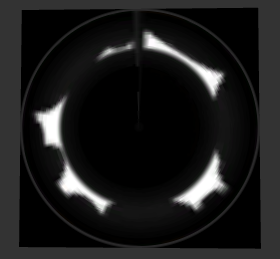Have a good dev!

5 Likes

this setup allows rotate (swizzle) and offset for flexibility

7 Likes

ThanksThis is really great:grinning: